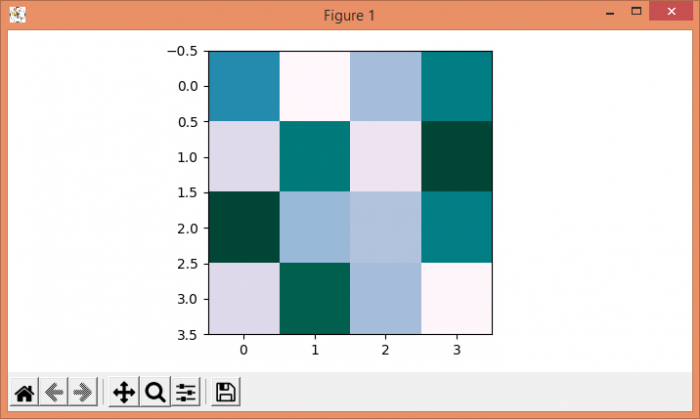# Colorplot of 2D array in Matplotlib

MatplotlibServer Side ProgrammingProgramming

To plot a colorplot of a 2D array, we can take the following steps −

• Create data (i.e., 2D array) using numpy.

• For colorplot, use imshow() method, with input data (Step 1) and colormap is "PuBuGn".

• To display the figure, use show() method.

## Example

import numpy as np
from matplotlib import pyplot as plt
plt.rcParams["figure.figsize"] = [7.00, 3.50]
plt.rcParams["figure.autolayout"] = True
data = np.random.rand(4, 4)
plt.imshow(data, cmap='PuBuGn')
plt.show()

## Output